﻿ G+--的平面性 The Planarity of G+--

Vol.07 No.03(2018), Article ID:24015,6 pages
10.12677/AAM.2018.73029

The Planarity of ${G}^{+--}$

Dan Wang1, Xiaoping Liu2

1College of Mathematics and System Sciences, Xinjiang University, Urumqi Xinjiang

2Xinjiang Institute of Engineering, Urumqi Xinjiang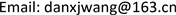Received: Feb. 22nd, 2018; accepted: Mar. 5th, 2018; published: Mar. 13th, 2018ABSTRACT

Let G be a simple graph. The transformation graph ${G}^{+--}$ of G is the graph with vertex set $V\left(G\right)\cup E\left(G\right)$ in which the vertex x and y are joined by an edge if and only if the following condition holds: 1) $x,y\in V\left(G\right)$ and x and y are adjacent in G, 2) $x,y\in E\left(G\right)$ , and x and y are not adjacent in G, 3) one of x and y is in V(G) and the other is in E(G), and they are not incident in G. In this paper, it is shown that G+−− is planar if and only if $|E\left(G\right)|\le 2$ or G is isomorphic to one of the following graphs: C3, C3 + K1, P4, P4 + K1, P3 + K2, P3 + K2 + K1, K1,3, K1,3 + K1, 3K2, 3K2 + K1, 3K2 + 2K1, C4, C4 + K1, 2P3.

Keywords:Total Graph, Planarity, Transformation Graph${G}^{+--}$ 的平面性

1新疆大学，数学与系统科学学院，新疆 乌鲁木齐

2新疆工程学院，新疆 乌鲁木齐1. 引言

2. 证明

$G\in \left\{{C}_{3},{C}_{3}+{K}_{1},{P}_{4},{P}_{4}+{K}_{1},{P}_{3}+{K}_{2},{P}_{3}+{K}_{2}+{K}_{1},{K}_{1,3},{K}_{1,3}+{K}_{1},3{K}_{2},3{K}_{2}+{K}_{1},3{K}_{2}+2{K}_{1}\right\}$ .

G+−−：3K2 + 2K1，P3 + K2 + K1，K1,3 + K1，C3 + K1，P4 + K1的变换图G+−−是可平面的。根据引理2.1，C3，P4，P3 + K2，K1,3，3K2，3K2 + K1的变换图G+−−也是可平面的。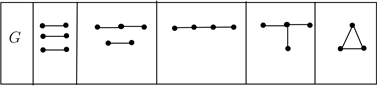Figure 1. All graphs of size 3 without isolated vertices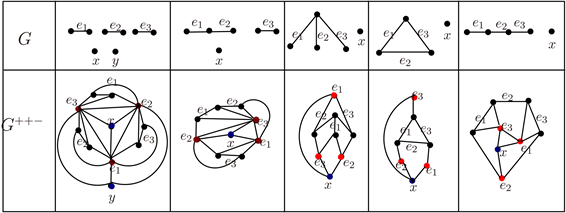Figure 2. Transformation graph G+−− of 3K2 + 2K1, P3 + K2 + K1, K1,3 + K1, C3 + K1, P4 + K1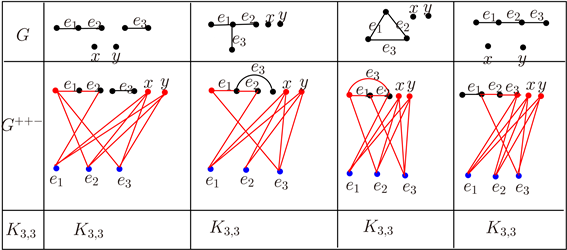Figure 3. Transformation graph G+−− of P3 + K2 + 2K1, K1,3 + 2K1, C3 + 2K1, P4 + 2K1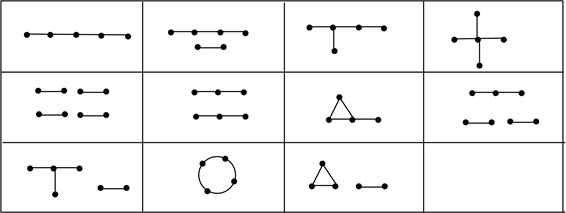Figure 4. All graphs of size 4 without isolated verticesFigure 5. Transformation graph of some graphs of size 4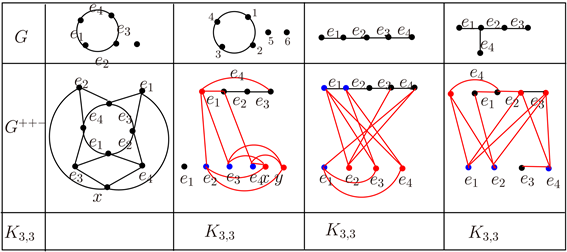Figure 6. Transformation graph of some graphs of size 4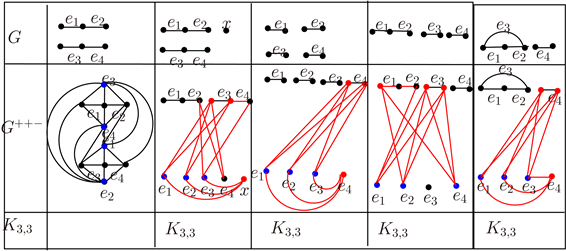Figure 7. Transformation graph of some graphs of size 4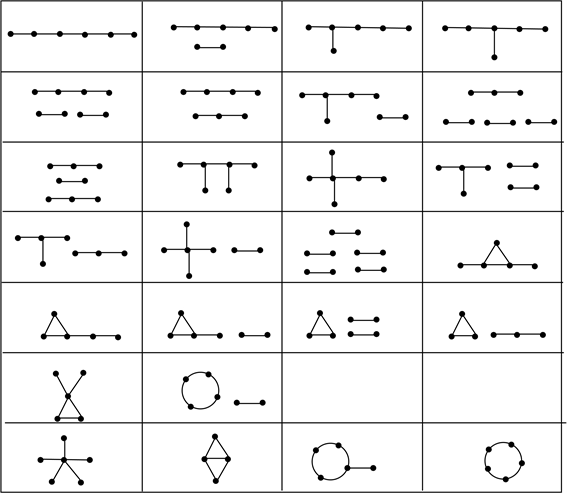Figure 8. All graphs of size 5 without isolated vertices

 1Bondy, J.A. and Murty, U.S.R. (1976) Graph Theory with Applications. Macmillan, London. https://doi.org/10.1007/978-1-349-03521-2

 Wu, B. and Meng, J. (2001) Basic Properties of Total Transformation Graphs. Journal of Mathematical Study, 34, 109-116.

 Behzad, M. (1967) A Criterion for the Planarity of the Total Graph of a Graph. Mathematical Proceedings of the Cambridge Philosophical Society, 63, 679-681. https://doi.org/10.1017/S0305004100041657

 Liu, X. (2006) On the Planarity of G−−−. Journal of Xinjiang University (Science & Engineering), 23, 159-161.

 Wu, B., Zhang, L. and Zhang, Z. (2005) The Trnasformation Graph Gxyz When x, y, z Î {+, −}. Discrete Mathematics, 296, 263-270. https://doi.org/10.1016/j.disc.2005.04.002

 Chen, J., Huang, L. and Zhou, J. (2012) Super Connectivity and Super Edge-Connectivity of Transformation Graphs G+−+. Ars Combinatoria, 105, 103-115.

 Deng, A. and Kelmans, A. (2017) Laplacian Spectra of Digraph Transformations. Linear and Multilinear Algebra, 65, 699-730. https://doi.org/10.1080/03081087.2016.1202183

 Deng, A., Feng, M. and Kelmans, A. (2016) Adjacency Polynomials of Digraph Transformations. Discrete Applied Mathematics, 206, 15-38. https://doi.org/10.1016/j.dam.2016.01.032

 Deng, A., Kelmans, A. and Meng, J. (2013) Laplacian Spectra of Regular Graph Transformations. Discrete Applied Mathematics, 161, 118-133. https://doi.org/10.1016/j.dam.2012.08.020

 Li, J. and Liu, J. (2014) Some Basic Properties of a Class of Total Transformation Digraphs. Ars Combinatoria, 116, 205-211.

 Xu, L. and Wu, B. (2008) Transformation Graph G−+−. Discrete Mathematics, 308, 5144-5148. https://doi.org/10.1016/j.disc.2007.09.040

 Yi, L. and Wu, B. (2009) The Transformation Graph G++−. Australasian Journal of Combinatorics, 44, 37-42.

 Zhen, L. and Wu, B. (2013) Hamiltonicity of Transformation Graph G+−−. Ars Combinatoria, 108, 117-127.